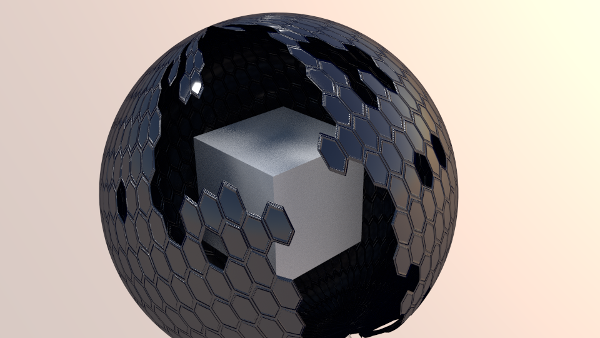## hexagonal animated texture

For this animation I used a similar technique as in my growing-texture animation. I created an image sequence in processing and used it as an animated image texture in blender. I used a transparent material everywhere the texture has a black pixel. The texture is also used as a displacement texture to make the hexagonal tiles better visible

The processing code is based on one of my abstract art generators I have written in processingThis is the processing code I used to create the texture

``````int [][] filled;
int rc = 21;
int cc = 61;

void setup() {
size(1770, 512);
smooth();
filled = new int[rc][cc];
int res[][] = new int[rc][cc];
for ( int r = 0; r<rc; r++) {
for ( int c = 0; c<cc; c++) {
res[r][c] = 255;
}
}

res[rc/2][cc/2] = 128+int(random(128));
filled = res;
}

void draw() {
background(0);
fill(255);
//  noStroke();
float s = 29.5/2;
float dr = sqrt( sq( 2*s ) - sq( s )) ;
float dc = 2 *s ;
for ( int r = 0; r<rc; r++) {
for ( int c = 0; c<cc; c++) {
fill( filled[r%(rc-1)][c % (cc-1)]);
if ( filled[r%(rc-1)][c % (cc-1)] < 255 ) {
stroke(128);
strokeWeight(5);

beginShape();
for ( int i=0; i<6; i++) {
vertex(  c*dc + s * cos( float(i)*TWO_PI/6 + PI/2 )+ ( r % 2 == 0  ? 0 : s), r*dr + s* sin( float(i)*TWO_PI/6 + PI/2 ) );
}
endShape( CLOSE );
stroke(255);
strokeWeight(1);

beginShape();
for ( int i=0; i<6; i++) {
vertex(  c*dc + s * cos( float(i)*TWO_PI/6 + PI/2 )+ ( r % 2 == 0  ? 0 : s), r*dr + s* sin( float(i)*TWO_PI/6 + PI/2 ) );
}
endShape( CLOSE );
}
}
}
filled = calc_fill( rc, cc);
println( frameCount );
if ( frameCount < 250 ) {
saveFrame( "hextex-####.png");
}
}

int[][] calc_fill( int rc, int cc ) {
int res[][] = filled;

for ( int r = 0; r<rc; r++) {
for ( int c = 0; c<cc; c++) {
if ( c > 0 && res[r][c-1] < 255 ||
c < cc-1 && res[r][c+1] < 255 ||
r > 0 && res[r-1][c] < 255 ||
r < rc-1 && res[r+1][c] < 255 ||
(r % 2 == 0) && r < rc-1 && c > 0 && res[r+1][c-1] < 255  ||
(r % 2 == 1) && r < rc-1 && c < cc-1 && res[r+1][c+1] < 255 ) {

if ( res[r][c] == 255 && random(100) > 92 ) {
res[r][c] = 128+int(random( 64 ));
}
}
}
}

return res;
}
``````